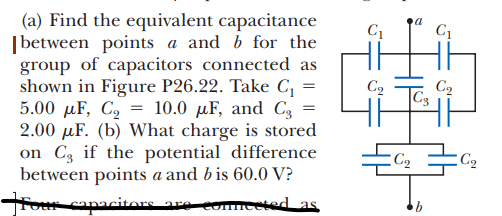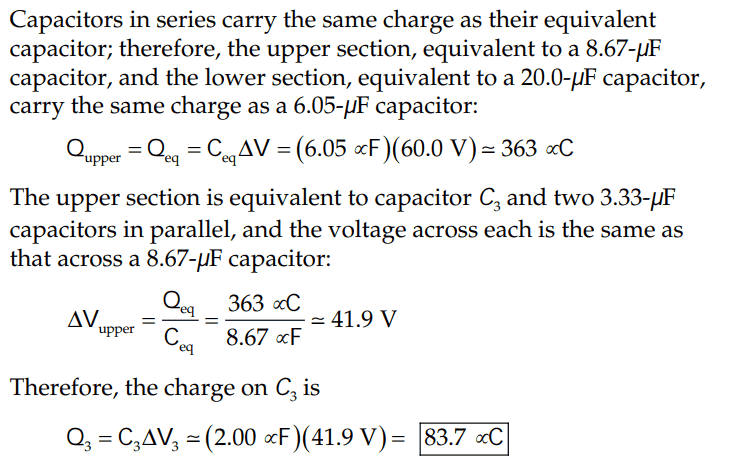# Finding charge on a capacitor given potential difference across two points

• ChiralSuperfields

#### ChiralSuperfields

Homework Statement
Relevant Equations
For this part(b) of this problem,The solution isHowever, I tried solving (b) like this:

Since ##Q_{total} = 363 \times 10^{-6} C## then ##Q_1 = 181.5 \times 10^{-6} C ## since the equivalent upper capacitor is in series with the equivalent bottom capacitor so should store the same amount of charge.

Since ##C_{upper} = 8.67 \times 10^{-6} C## then voltage across upper equivalent capacitor is ##\frac {181.5}{8.67} = 21V ## then charged stored by ##C_3## is ##Q_3 = 2 \times 10^{6} \times 21 = 4.2 \times 10^{-5} ##

I don't understand why they use the total charge for the upper capacitors when they only store half the charge.

Many thanks!

What do you label as Q1?

•ChiralSuperfields
What do you label as Q1?

I'm not sure, just some notation for the upper equivalent capacitors charge.

Then this is already labeled as Q total. This is the charge on the upper group of capacitors. And the same charge is on the lower group, which is in series with the upper group.
.

•ChiralSuperfields
Then this is already labeled as Q total. This is the charge on the upper group of capacitors. And the same charge is on the lower group, which is in series with the upper group.
.
Thank you for your reply @nasu! I forgot capacitor in series have the same charge!